Courses

# Ex 1.2 NCERT Solutions - Real Numbers Class 10 Notes | EduRev

## Class 10 : Ex 1.2 NCERT Solutions - Real Numbers Class 10 Notes | EduRev

The document Ex 1.2 NCERT Solutions - Real Numbers Class 10 Notes | EduRev is a part of the Class 10 Course Class 10 Mathematics by VP Classes.
All you need of Class 10 at this link: Class 10

Exercise 1.2
Ques 1: Express each number as a product of its prime factors:
(i) 140

(ii) 156
(iii) 3825
(iv) 5005
(v) 7429
Sol: (i) Using factor tree method, we have: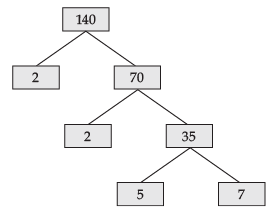∴ 140 = 2 × 2 × 5 × 7
= 22 × 5 × 7
(ii) Using factor tree method, we have: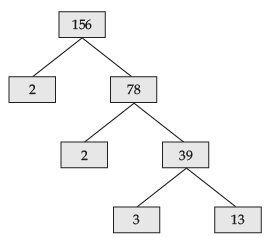(ii) 156 = 2 x 2 x 3 x 13
= 22 x 3 x 13
(iii) Using factor tree method, we have: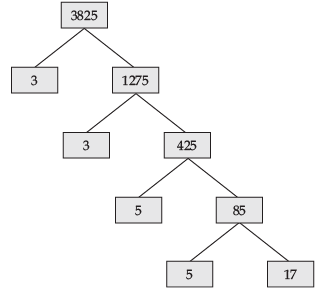3825 = 3 × 3 × 5 × 5 × 17
= 32 × 52 × 17
(iv) Using factor tree method, we have: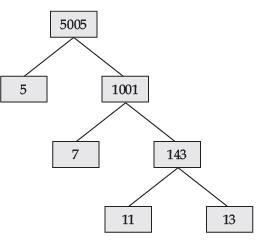∴ 5005 = 5 × 7 × 11 × 13
(v) Using the factor tree method, we have: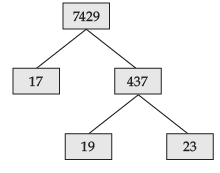∴  7429 = 17 × 19 × 23

Ques 2: Find the LCM and HCF of the following pairs of integers and verify that LCM × HCF = product of the two numbers.
(i) 26 and 91

(ii) 510 and 92
(iii) 336 and 54
Sol: (i) Using factor-tree method, we have: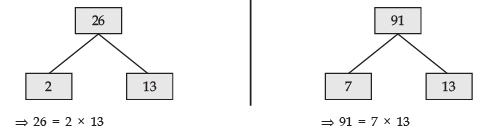∴ LCM of 26 and 91 = 2 × 7 × 13 = 182
HCF of 26 and 91 = 13
Now, LCM × HCF = 182 × 13 = 2366
and 26 × 91 = 2366
i.e.,  LCM × HCF = Product of two numbers.
(ii) Using the factor tree method, we have: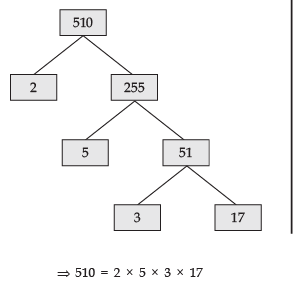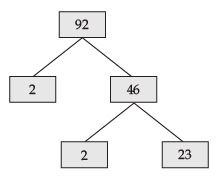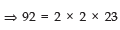∴ LCM of 510 and 92 = 2 × 3 × 5 × 17 × 2 × 23
= 23460
HCF of 510 and 92 = 2
⇒ LCM × HCF  = 23460 × 2 = 46920
510 × 92 = 46920
i.e., LCM × HCF = Product of two numbers.
(iii) Using the factor tree method, we have: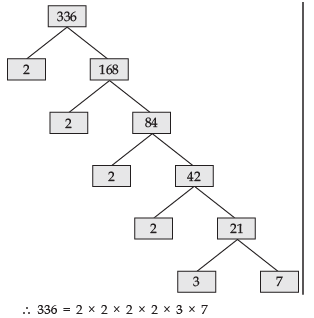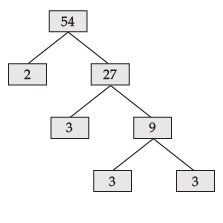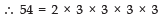LCM of 336 and 54 = 2 × 2 × 2 × 2 × 3 × 3 × 3 × 7 = 3024
HCF of 336 and 54 = 2 × 3 = 6
LCM × HCF = 3024 × 6 = 18144
Also 336 × 54 = 18144
Thus, LCM × HCF  = Product of the numbers

Ques 3: Find the LCM and HCF of the following integers by applying the prime factorisation method.
(i) 12, 15 and 21
(ii) 17, 23 and 29
(iii) 8, 9 and 25
Sol. (i) We have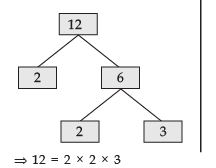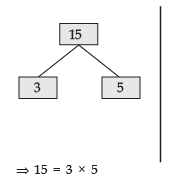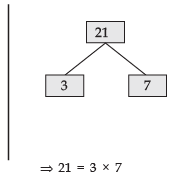∴  HCF of 12, 15 and 21    is 3
LCM of 12, 15 and 21    is 2 × 2 × 3 × 5 × 7 or 420
(ii) We have:

 17 = 1 × 17 ⇒ HCF of 17, 23 and 29 is 1 23 = 1 × 23 LCM of 17, 23 and 29 29 = 1 × 29 = 17 × 23 × 29= 11339

(iii) We have: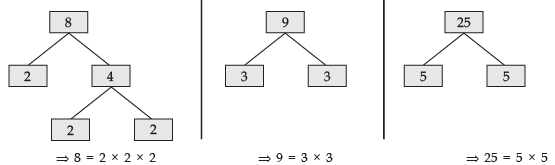∴ HCF of 8, 9 and 25 is  1
LCM of 8, 9 and 25  is  2 × 2 × 2 × 3 × 3 × 5 × 5 or 1800

Ques 4: Given that HCF (306, 657) = 9, find LCM (306, 657).
Sol: Here, HCF of 306 and 657 = 9
We have:
LCM × HCF =  Product of the numbers
∴ LCM × 9 = 306 × 657
⇒ LCM = 34 × 657/9
= 22338
Thus, LCM of 306 and 657 is 22338.

Ques 5: Check whether 6n can end with the digit 0 for any natural number n.
Sol: Here, n is a natural number and let 6n end in 0.
∴ 6n is divisible by 5.
But the prime factors of 6 are 2 and 3.
⇒ 6n = (2 × 3)n
i.e., In the prime factorisation of 6n, there is no factor 5.
Since, by the Fundamental Theorem of Arithmetic, every composite number can be expressed as a product of primes and this factorisation is unique apart from the order in which the prime factorisation occurs.
∴ Our assumption that 6n ends in 0, is wrong.

Ques 6: Explain why 7 × 11 × 13 + 13 and 7 × 6 × 5 × 4 × 3 × 2 × 1 + 5 are composite numbers.
Sol:
We have 7 × 11 × 13 + 13
= 13 [(7 × 11) + 1]
= 13 
i.e., 13 × 78 cannot be a prime number because it has a factor 13.
∴ 13 × 78 is a composite number.
Also 7 × 6 × 5 × 4 × 3 × 2 × 1 + 5
= 5 [7 × 6 × 4 × 3 × 2 × 1 + 1]
which is also not a prime number.    [∵ It has a factor 5]
Thus, ‘7 × 11 × 13 + 13’ and ‘7 × 6 × 5 × 4 × 3 × 2 × 1 + 5’ are composite numbers.

Ques 7: There is a circular path around a sports field. Sonia takes 18 minutes to drive one round of the field, while Ravi takes 12 minutes for the same. Suppose they both start at the same point and at the same time, and go in the same direction. After how many minutes will they meet again at the starting point?
Sol:
Time taken by Sonia to drive one round of the field = 18 minutes.
Time is taken by Ravi to arrive one round of the field = 12 minutes.
The L.C.M. of 18 and 12 gives the exact number of minutes after which they meet at the starting point again.
We have: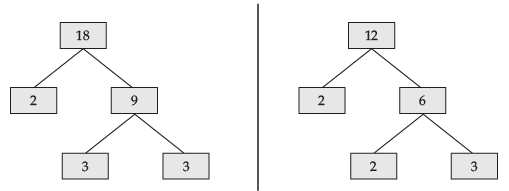⇒ 18 = 2 × 3 × 3  and 12 = 2 × 2 × 3
∴  LCM of 18 and 12 = 2 × 2 × 3 × 3 = 36
Thus, they will meet again at the starting point after 36 minutes.

Revisiting Irrational numbers
A number is said to be an irrational number, if it cannot be expressed in the form p/q, where ‘p’ and ‘q’ are integers and q ≠ 0.
For example,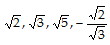, 0.10110111011110 ..., p, etc., are irrational.

Offer running on EduRev: Apply code STAYHOME200 to get INR 200 off on our premium plan EduRev Infinity!

132 docs

,

,

,

,

,

,

,

,

,

,

,

,

,

,

,

,

,

,

,

,

,

;